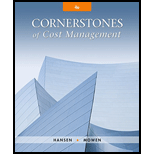# Kumar, Inc., evaluates managers of producing departments on their ability to control costs. In addition to the costs directly traceable to their departments, each production manager is held responsible for a share of the costs of a support center, the Human Resources (HR) Department. The total costs of HR are allocated on the basis of actual direct labor hours used. The total costs of HR and the actual direct labor hours worked by each producing department are as follows: *$0.25 per direct labor hour plus$100,000. Required: 1. Allocate the HR costs to each producing department for Year 1 and Year 2 using the direct method with actual direct labor hours and actual HR costs. 2. Discuss the following statement: “The costs of human resource-related matters increased by 25 percent for Department A and decreased by over 16 percent for Department B. Thus, the manager of Department B must be controlling HR costs better than the manager of Department A.” 3. Can you think of a way to allocate HR costs so that a more reasonable and fair assessment of cost control can be made? Explain.### Cornerstones of Cost Management (C...

4th Edition
Don R. Hansen + 1 other
Publisher: Cengage Learning
ISBN: 9781305970663

#### Solutions

Chapter
Section### Cornerstones of Cost Management (C...

4th Edition
Don R. Hansen + 1 other
Publisher: Cengage Learning
ISBN: 9781305970663
Chapter 7, Problem 18E
Textbook Problem
1048 views

## Kumar, Inc., evaluates managers of producing departments on their ability to control costs. In addition to the costs directly traceable to their departments, each production manager is held responsible for a share of the costs of a support center, the Human Resources (HR) Department. The total costs of HR are allocated on the basis of actual direct labor hours used. The total costs of HR and the actual direct labor hours worked by each producing department are as follows:*$0.25 per direct labor hour plus$100,000.Required: 1. Allocate the HR costs to each producing department for Year 1 and Year 2 using the direct method with actual direct labor hours and actual HR costs. 2. Discuss the following statement: “The costs of human resource-related matters increased by 25 percent for Department A and decreased by over 16 percent for Department B. Thus, the manager of Department B must be controlling HR costs better than the manager of Department A.” 3. Can you think of a way to allocate HR costs so that a more reasonable and fair assessment of cost control can be made? Explain.

1.

To determine

Allocate the HR costs to each producing department for year 1 and Year 2 by using the method with actual direct labor hours and actual HR costs.

### Explanation of Solution

Cost Allocation: The process of assigning the cost to the cost object is cost allocation. The cost allocation is done by the accountant to allocate the manufacturing overhead cost to different divisions. The allocation base for ascertaining the charging rates is based upon on the budgeted amounts and not actual amounts.

Compute allocation ratios:

 Year 1 Year 2 Department A (1)0.4 (2)0.5 Department B (3)0.6 (4)0.5

Table (1)

Compute allocation of costs:

 Year 1 Year 2 Department A (5)$48,000 (6)$60,000 Department B (7)$72,000 (8)$60,000

Table (2)

Working notes:

(1)Calculate the allocation ratio for department A for the year 1:

Allocation ratio for department A for the year 1}=DirectlaborhoursworkedforDepartmentA(DirectlaborhoursworkedforDepartmentA+DirectlaborhoursworkedforDepartmentB)=24,00024,000+36,000=24,00060,000=0.4

(2)Calculate the allocation ratio for department A for the year 2:

Allocation ratio for department A for the year 2}=DirectlaborhoursworkedforDepartmentA(DirectlaborhoursworkedforDepartmentA+DirectlaborhoursworkedforDepartmentB)=25,00025,000+32,000=25,00050,000=0.5

(3)Calculate the allocation ratio for department B for the year 1:

Allocation ratio for department B for the year 1}=DirectlaborhoursworkedforDepartmentB(DirectlaborhoursworkedforDepartmentA+DirectlaborhoursworkedforDepartmentB)=36,00024,000+36,000=36,00060,000=0

2.

To determine

Discuss the statement.

3.

To determine

Suggest a way to allocate HR costs in a more reasonable way and explain the manner in which fair assessment of cost control can be made.

### Still sussing out bartleby?

Check out a sample textbook solution.

See a sample solution

#### The Solution to Your Study Problems

Bartleby provides explanations to thousands of textbook problems written by our experts, many with advanced degrees!

Get Started

Find more solutions based on key concepts
Discuss target market strategies

MKTG 12:STUDENT ED.-TEXT

What is bolt-on software?

Accounting Information Systems

Why are there so many laws relating to HRM practices?

Foundations of Business (MindTap Course List)

Describe the transactions recorded in the following T accounts:

College Accounting (Book Only): A Career Approach

Why is EBIT generally considered independent of financial leverage? Why might EBIT actually be affected by fina...

Fundamentals of Financial Management, Concise Edition (with Thomson ONE - Business School Edition, 1 term (6 months) Printed Access Card) (MindTap Course List)